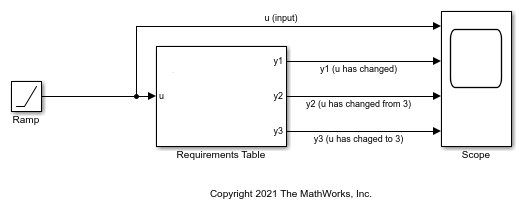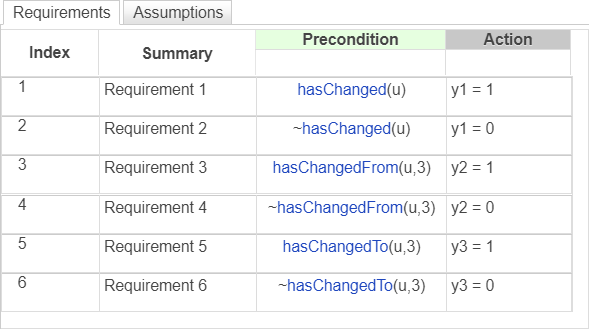## Detect Data Changes by Using Requirements Table Blocks

Requirements Table blocks can detect changes in the values of data between time steps. You can use change detection operators to determine when data changes to or from a value.

### Change Detection Operators

To detect changes in data, use the operators listed in this table.

OperatorSyntaxDescription
`hasChanged``tf = hasChanged(data_name)`Returns `1` (`true`) if the value of `data_name` at the beginning of the current time step is different from the value of `data_name` at the beginning of the previous time step. Otherwise, the operator returns `0` (`false`).
`hasChangedFrom``tf = hasChangedFrom(data_name,value)`Returns `1` (`true`) if the value of `data_name` was equal to the specified `value` at the beginning of the previous time step and is a different value at the beginning of the current time step. Otherwise, the operator returns `0` (`false`).
`hasChangedTo``tf = hasChangedTo(data_name,value)`Returns `1` (`true`) if the value of `data_name` was not equal to the specified `value` at the beginning of the previous time step and is equal to `value` at the beginning of the current time step. Otherwise, the operator returns `0` (`false`).

The input argument `data_name` is data defined in the Requirements Table block, specified as a:

• Scalar

• Matrix or an element of a matrix

• Structure or a field in a structure

• Valid combination of structure fields or matrix elements

For the `hasChangedFrom` and `hasChangedTo` operators, the argument `value` must be an expression that resolves to a value that is comparable with `data_name`. For example, if `data_name` is a matrix, then `value` must resolve to a matrix value with the same dimensions as `data_name`.

### Example of Requirements Table Block with Change Detection

This example shows how the operators `hasChanged`, `hasChangedFrom`, and `hasChangedTo` detect specific changes in an input signal. In this example, a Ramp (Simulink) block sends a discrete, increasing time signal to a Requirements Table block.The model uses a fixed-step solver with a step size of `1`. The signal increments by `1` every time step. The block checks the input `u` for these changes:

• Changes from the previous time step

• A change from the value `3`

• A change to the value `3`

To check the signal, the block calls three change detection operators and specifies six requirements. Each change detection operator determines the value of the output data `y1`, `y2`, and `y3`.

• If `hasChanged(u)` is `true`, `y1` equals `1`. Otherwise, `y1` equals `0`.

• If `hasChangedFrom(u,3)` is `true`, `y2` equals `1`. Otherwise, `y2` equals `0`.

• If `hasChangedTo(u,3)` is `true`, `y3` equals `1`. Otherwise, `y3` equals `0`.During simulation, the Scope (Simulink) block shows the input and output signals for the block.

• The value of `u` increases by `1` every time step.

• The value of `y1` changes from `0` to `1` at time `t = 1`. The value of `y1` remains `1` because `u` continues to change at each subsequent time step.

• The value of `y2` changes from `0` to `1` at time `t = 4` when the value of `u` changes from `3` to `4`. The value of `y2` returns to `0` after one time step.

• The value of `y3` changes from `0` to `1` at time `t = 3` when the value of `u` changes from `2` to `3`. The value of `y3` returns to `0` after one time step.